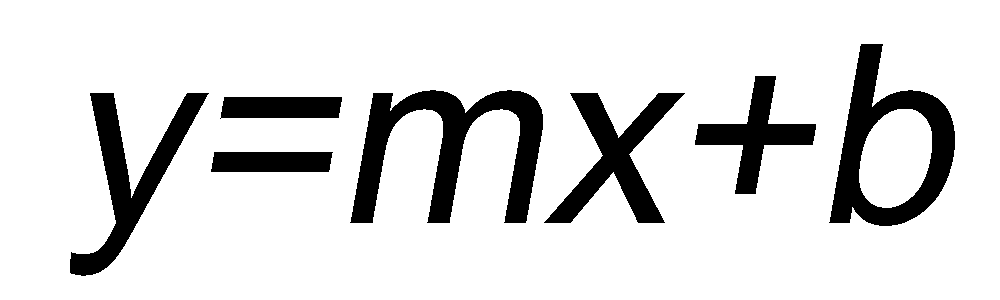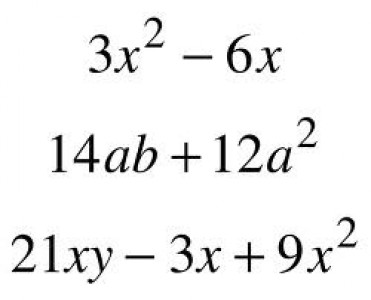# Difference between Algebraic Expressions and Equations

Algebraic expression and equation are two terms used in algebra, and are often confused by students who consider them interchangeable. However, both algebraic expression and equation are two distinct algebraic terms that can easily be differentiated from each other. The basic and the most obvious difference between the two is the equality sign (=). An equation is always written with an equality sign separating two mathematical or algebraic terms. An algebraic expression, on the other hand, is an algebraic statement that contains no equality sign.

The term expression used in mathematics or algebra is equivalent to an English phrase, just delivering the information, without relating it to any other number or algebraic term. An equation, on the other hand, is equivalent to an English sentence, delivering mathematical information by comparing and contrasting different terms with an equality or inequality sign between them. Furthermore, an algebraic expression is always simplified, while an equation can be simplified as well as solved, to find a definite value for some variable.

An equation always has a relation symbol, whereas an algebraic expression has no relationship symbol. For example, ‘Ten is five less than a number’ written algebraically as 10 = x – 5 , and ‘a number is less than five’ written algebraically as x < 5, are equations; whereas, a number less than five x or five less than a number x – 5, are examples of algebraic expressions.

### Instructions

• 1

Equation:

In algebra, an equation is an expression of the shape A = B, where A and B are algebraic expressions with one or more unknown variables. Students often confuse an algebraic equation with equality, but they are two distinct terminologies. An equality is a mathematical statement that states that the expression on the left hand side, when simplified, should be equal to the expression on the right hand side, and it does not involve any unknown variable. An equation, on the other hand , is not a statement; rather, it is a problem comprising one or more unknown variables, whose values, called solutions, are to be determined on the basis of the relationship given in the equation. For example, 3x + 5 = 2 is an equation, with one unknown “x”.

Image courtesy: serc.carleton.edu• 2

Algebraic expression:

An algebraic expression, in mathematics, is an expression consisting of constants, variables and a finite number of algebraic operations.

Image courtesy: docs.exdat.com•